# Parabola Help

#### MissTrinity

##### New member
This is the question I've encountered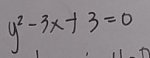I have managed to get this form x= y2/3 +1
I have checked the graph from both forms and the vertex lies on (1,0)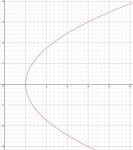(as shown in this graph)

The part which I am confused at is how was the vertex was solved, I am very familiar of this form y=ax2+bx+c and yet as I saw the equation I began to get confused and couldn't use the formulas used to find vertex though I tried severely here is what I got
This is my entire solution, but please do ignore x=4 since this is calculus problem though I am much concern on how to graph this new equation I just encountered, it just felt new to me i haven't encountered or recall encountering this back in analytic geometry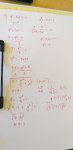(i tried, im confused still)

Thank you so much and hope to get a reply soon, it will be a big help

#### Dr.Peterson

##### Elite Member
This is the question I've encountered
View attachment 11007

I have managed to get this form x= y2/3 +1
I have checked the graph from both forms and the vertex lies on (1,0)

The part which I am confused at is how was the vertex was solved, I am very familiar of this form y=ax2+bx+c and yet as I saw the equation I began to get confused and couldn't use the formulas used to find vertex though I tried severely here is what I got
This is my entire solution, but please do ignore x=4 since this is calculus problem though I am much concern on how to graph this new equation I just encountered, it just felt new to me i haven't encountered or recall encountering this back in analytic geometry
(i tried, im confused still)
Are you confused because x and y have been swapped from the form you know? Just swap x and y in your thinking.

The form x = y2/3 + 1 tells you the vertex: this is the function x = y2, compressed by a factor of 1/3 in the x direction, and shifted 1 unit in the positive x direction, just as y = (1/3)x2 + 1 would be a vertical parabola compressed vertically by a factor of 1/3, and shifted up 1.

Or, if you are familiar with the formula x = -b/(2a) for the x-coordinate of the vertex of y=ax2+bx+c, the formula for the y-coordinate of x=ay2+by+c is y = -b/(2a). Do you see how I have just swapped x and y?

#### Harry_the_cat

##### Senior Member
This is the question I've encountered
View attachment 11007

I have managed to get this form x= y2/3 +1
I have checked the graph from both forms and the vertex lies on (1,0)
View attachment 11008(as shown in this graph)

The part which I am confused at is how was the vertex was solved, I am very familiar of this form y=ax2+bx+c and yet as I saw the equation I began to get confused and couldn't use the formulas used to find vertex though I tried severely here is what I got
This is my entire solution, but please do ignore x=4 since this is calculus problem though I am much concern on how to graph this new equation I just encountered, it just felt new to me i haven't encountered or recall encountering this back in analytic geometry
View attachment 11009(i tried, im confused still)

Thank you so much and hope to get a reply soon, it will be a big help
Does it help if you know that the graph of $$\displaystyle x=\frac{y^2}{3}+1$$ is the same as the graph of $$\displaystyle y=\frac{x^2}{3}+1$$ except all the x's and y's have interchanged.
Graphically, that means one graph is reflected in the diagonal line y=x to get the other.

So of the vertex of $$\displaystyle y=\frac{x^2}{3}+1$$ is (0,1) , which can be found in various usual ways, then the vertex of the other is (1,0).

#### MissTrinity

##### New member
Are you confused because x and y have been swapped from the form you know? Just swap x and y in your thinking.

Or, if you are familiar with the formula x = -b/(2a) for the x-coordinate of the vertex of y=ax2+bx+c, the formula for the y-coordinate of x=ay2+by+c is y = -b/(2a). Do you see how I have just swapped x and y?
Yes unfortunate I do get confused in swapping x and y but I did try it again following x=ay2+by+c is y = -b/(2a)
here is what I got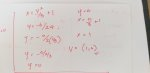thank you very much for your help

#### Jomo

##### Elite Member
This is the question I've encountered
View attachment 11007

I have managed to get this form x= y2/3 +1
I have checked the graph from both forms and the vertex lies on (1,0)
View attachment 11008(as shown in this graph)

The part which I am confused at is how was the vertex was solved, I am very familiar of this form y=ax2+bx+c and yet as I saw the equation I began to get confused and couldn't use the formulas used to find vertex though I tried severely here is what I got
This is my entire solution, but please do ignore x=4 since this is calculus problem though I am much concern on how to graph this new equation I just encountered, it just felt new to me i haven't encountered or recall encountering this back in analytic geometry
View attachment 11009(i tried, im confused still)

Thank you so much and hope to get a reply soon, it will be a big help
In your 1st attempt you did not solve for x correctly. In your 2nd attempt you did not solve for y2 correctly. You also wrote that y2 = 9 and concluded that y = 3. This is clearly not correct. Please fix this.

Last edited:

#### MissTrinity

##### New member
In your 1st attempt you did not solve for x correctly. In your 2nd attempt you did not solve for y2 correctly. You also wrote that y2 = 9 and concluded that y = 3. This is clearly not correct. Please fix this.
I'm clearly aware it is wrong and with the help of the of Dr. Peterson, i did get to answer it right and followed the same procedure to the rest of my calculus assignment in working with curves in this case parabola, I've checked my own graph to a computer generated graph so i could verify my solution.problem solved

#### MissTrinity

##### New member
You also wrote that y2 = 9 and concluded that y = 3. This is clearly not correct. Please fix this.
I just got genuinely confused at this part you said.
if you checked this part of my solution (which again if you view the whole solution I shared it is for most part wrong especially in solving for the vertex)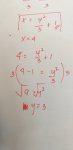in here I was solving for the intersection between the curve and the line x=4, in which I did get right using the equation x= y2/3 +1, but i know you could still use the given equation I just happen to check if I would still end up in the right direction then did the math
Isn't it indeed the fact y2=9 if y=3 ?
also just to share that for the equationwith the line x=4, they intersect at the coordinates x=4 & y=3
shown here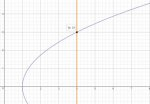thank you for pointing out the wrong parts of my solution which was already indeed obvious, isn't that why I asked for help? a guidance? if you however commented ahead before this gentlemen did, I would have been looking at my calculator even more confused just to answer what is y2=9 if y=3? or if i did my simplification by doing the square root solving right

#### Jomo

##### Elite Member
I just got genuinely confused at this part you said.
if you checked this part of my solution (which again if you view the whole solution I shared it is for most part wrong especially in solving for the vertex)
View attachment 11013
in here I was solving for the intersection between the curve and the line x=4, in which I did get right using the equation x= y2/3 +1, but i know you could still use the given equation I just happen to check if I would still end up in the right direction then did the math
Isn't it indeed the fact y2=9 if y=3 ?
also just to share that for the equation View attachment 11014 with the line x=4, they intersect at the coordinates x=4 & y=3
shown here
View attachment 11015

thank you for pointing out the wrong parts of my solution which was already indeed obvious, isn't that why I asked for help? a guidance? if you however commented ahead before this gentlemen did, I would have been looking at my calculator even more confused just to answer what is y2=9 if y=3? or if i did my simplification by doing the square root solving right
y can also be -3.

#### MissTrinity

##### New member
y can also be -3.
i see but it would be positive 3 for my solution yes? all according from those i have solved and shared

#### Jomo

##### Elite Member
i see but it would be positive 3 for my solution yes? all according from those i have solved and shared
Please check for yourself. Is (4,3) and (4,-3) on your graph or do they satisfy your equation????